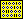1. BVB - BibliotheksVerbund Bayern Solr-Zugang
 1 Handbook of mathematical functions ; with formulas, graphs, and mathematical tables / 1972 2 Handbook of mathematical functions ; with formulas, graphs, and mathematical tables / 1965 3 Handbook of mathematical functions ; with formulas, graphs, and mathematical tables / 1972 4 Handbook of mathematical functions ; With formulas, graphs, and mathematical tables / 1972 5 Handbook of mathematical functions ; With formulas, graphs, and mathematical tables / 1969 6 Handbook of mathematical functions ; with formulas, graphs and mathematical tables / 1972 7 Handbook of mathematical functions ; with formulas, graphs, and mathematical tables / 1968 8 Handbook of mathematical functions ; with formulas, graphs and mathematical tables / 1968 9 Handbook of mathematical functions ; with formulars, graphs, and mathematical tables / 1972 10 Handbook of mathematical functions ; with formulas, graphs, and mathematical tables / 1970 11 Handbook of mathematical functions ; with formulas, graphs, and mathematical tables / 1970
 Total Number of Matches: 11 Time needed: 0.658 seconds

 2. German National Library of Science and Technology (TIB)
 Total Number of Matches: 1 Time needed: 0.622 seconds

 3. IDS Bale/Berne
 Total Number of Matches: 2 Time needed: 0.622 seconds

 4. Union Catalog Austria
 Total Number of Matches: 1 Time needed: 0.635 seconds

 5. Staatsbibliothek zu Berlin
 Total Number of Matches: 1 Time needed: 0.657 seconds

 6. Union Catalogue of Swedish Libraries LIBRIS
 Total Number of Matches: 2 Time needed: 0.679 seconds

 7. Amazon.de - English Books
 Total Number of Matches: 1 Time needed: 0.674 seconds

 8. HeBIS, Union Catalog Hesse
 Total Number of Matches: 6 Time needed: 0.856 seconds

 9. SWB, Union Catalog Southwest Germany
 Total Number of Matches: 7 Time needed: 0.919 seconds

 10. SUDOC, French Union Catalog
 Total Number of Matches: 1 Time needed: 0.939 seconds

 11. ZVAB - Catalogue of antiquarian Books
 Total Number of Matches: 1 Time needed: 0.501 seconds

 12. oria.no - BIBSYS
 1 Handbook of mathematical functions : / 1965
 Total Number of Matches: 1 Time needed: 1.038 seconds

 13. GBV, Union Catalog Northern Germany
1. Handbook of mathematical functions : with formulas, graphs, and mathematical tables / Abramowitz, Milton. - 9. Dover print.; [Nachdr. der Ausg. von 1972]. - New York, NY : Dover Publ., 2013
2. Handbook of Mathematical Functions : with Formulas, Graphs, and Mathematical Tables / Abramowitz, Milton. - 9. Dover print.; [Nachdr. der Ausg. von 1972]. - New York : Dover Publ., 20123. Handbook of mathematical functions : with formulas, graphs, and mathematical tables / Abramowitz, Milton. - 9. Dover print.; [Nachdr. der Ausg. von 1972]. - New York, NY : Dover Publ., [ca. 2007]
4. Handbook of mathematical functions : with formulas, graphs, and mathematical tables / Abramowitz, Milton. - 9. Dover printing. - New York : Dover Publ., [1973?]
5. Handbook of mathematical functions : with formulas, graphs, and mathematical tables / Abramowitz, Milton. - 9. Dover pr. - New York : Dover Publ., circa 1972
6. Handbook of mathematical functions : with formulas, graphs, and mathematical tables / Abramowitz, Milton. - 10. print. - New York, NY : Dover, 1972
7. Handbook of mathematical functions : with formulas, graphs, and mathematical tables / Abramowitz, Milton. - New York : Dover Publications, 1972
8. Handbook of mathematical functions : with formulas, graphs, and mathematical tables / Abramowitz, Milton. - 8. Dover print., with corr.; [Reprint of NBS ed.]. - New York, NY : Dover Publ., 1972
9. Handbook of mathematical functions with formulas, graphs, and mathematical tables / Abramowitz, Milton. - 10. print., Dec. 1972, with corr. - Washington, DC : U.S. Gov. Print. Off., 1972
10. Handbook of mathematical functions : with formulas, graphs, and mathematical tables / Abramowitz, Milton. - 7. Dover print.; [Reprint of NBS ed.] 9. print., Nov. 1970. - New York, NY : Dover Publ., 
 More Matches...
 Total Number of Matches: 16 Time needed: 1.243 seconds

 14. British Union Catalog - COPAC
 Total Number of Matches: 8 Time needed: 3.626 seconds

 Status
 Fragen und Anmerkungen an: Uwe Dierolf (dierolf@ubka.uni-karlsruhe.de) Preise und Auszeichnungen:Pressestimmen zum KVK